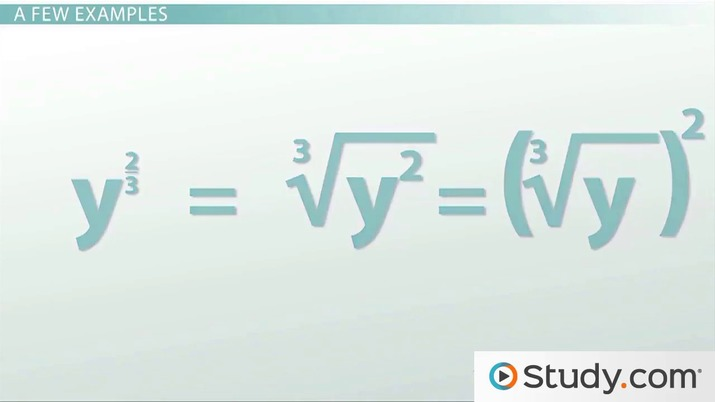# Rational Exponents: Overview & Examples

Maria Airth, Kathryn Maloney
• Author
Maria Airth

Maria has taught University level psychology and mathematics courses for over 20 years. They have a Doctorate in Education from Nova Southeastern University, a Master of Arts in Human Factors Psychology from George Mason University and a Bachelor of Arts in Psychology from Flagler College.

• Instructor
Kathryn Maloney

Kathryn teaches college math. She holds a master's degree in Learning and Technology.

Learn about rational exponents. Study the definition of rational exponents, learn how to solve them, and work through examples of rational exponent equations. Updated: 10/06/2021

Show

## What is a Rational Exponent?

Before exploring rational exponents, it is necessary to understand rational numbers. A rational number is any number that can be expressed as a fraction. Even whole numbers, integers, are part of the rational number group because we can express any integer as a fraction by setting it over the number one.

Here are some examples of rational numbers:

Number Fractional Form
1.5 3/2
4 4/1
0.01 1/100
0.25 1/4

Any number that can be expressed as a fraction is considered a rational number; however, in practice, rational numbers refer to fractions.

What is a rational exponent? A rational exponent occurs when a base number is being raised to a fractional (or rational) power. To better understand the rational definition of exponents and prepare for this lesson, review these keywords associated with these two numbers:

{eq}4^{\frac{1}{3}} and \sqrt{4} {/eq}

Term Definition Example from Above
Base Main number being manipluated 4
Exponent Superscripted power of the base 1/3
Rational Exponent Exponent in fraction form 1/3
Radical form A number using a root symbol
Root number (index) Superscripted number outside the root symbol 3
Radicand Base number in a radical number (under the root symbol) 4

Understanding the parts of problems using rational exponents is the first step to solving them.An error occurred trying to load this video.

Try refreshing the page, or contact customer support.

Coming up next: Simplifying Expressions with Rational Exponents

### You're on a roll. Keep up the good work!

Replay
Your next lesson will play in 10 seconds
• 0:06 Quick Review
• 1:20 Examples
• 3:03 Lesson Summary
Save Save

Want to watch this again later?

Timeline
Autoplay
Autoplay
Speed Speed

## Solving Rational Exponents

Solving rational exponents is a matter of rewriting the rational exponent in radical form using these steps:

1. Make the numerator of the original rational exponent the new exponent of the base.
2. Write the base on the (new) exponent as the radicand (number under the root symbol).
3. Make the denominator of the original rational exponent the root number, called the index of the radical.
4. Simplify the radicand expression by expanding the exponent (if possible).
5. Simplify the radical (if possible).For example, if the rational exponent was {eq}\frac{3}{2} {/eq}, then the radical form of this number would require the square root of a cubed number (2 outside the radical and a 3 as the exponent inside the radical).

## Rational Exponent Equation Examples

The best way to understand how to do something is to work through it. Try these rational exponent equations.

### Example 1: Rational Exponent Equation Example

Simplify: {eq}5^{\frac{1}{2}} {/eq}

Step 1
Identify the 1 as the new exponent.
Step 2
Identify the base as the radicand, 5.
Step 3
Identify the index number as 2.

So, the rational number {eq}5^{\frac{1}{2}} {/eq} can be rewritten as {eq}\sqrt{{5}^1} {/eq}

Step 4
An exponent of 1 is already as simple as possible and does not need to be explicitly written.
Step 5
The square root of 5 is not an integer nor a rational number, thus it can be left as a radical or changed to a decimal rounded to any place value desired.

Final Answer: {eq}5^{\frac{1}{2}} = \sqrt{5} {/eq} or {eq}2.24 {/eq} (rounded to the hundredth place value).

### Example 2: Rational Exponent Equation Example

Simplify: {eq}(2m)^{\frac{4}{3}} {/eq}

Following steps 1 through 3, rewrite the number with the numerator, 4, as the exponent of the radicand/base, 2, using an index of 3 on the radical.

{eq}\sqrt{2m^4} {/eq}

Step 4
Simplify the exponent by calculating {eq}2\times2\times2\times2=16 {/eq}.
Step 5
Simplify the radical: 16 is not a perfect cube, however {eq}m^4 {/eq} can be simplified in this instance.

### Example 3: Rational Exponent Equation Example

Work backward to rewrite this radical expression as a rational exponent expression: {eq}(\sqrt{x})^3 {/eq}

Step 1
Start inside the parenthesis and identify that the exponent for the radicand is 1.
Step 2
Identify that the index is 5.
Step 3
Rewrite the radical expression as the rational exponent expression of {eq}x^{\frac{1}{5}} {/eq}.
Step 4
Remember that when a power is raised to a power the two powers are multiplied: {eq}\frac{1}{5} \times 3=\frac{3}{5} {/eq}
Step 5

### Example 4: Rational Exponent Equation Example

Rewrite this radical expression as a rational exponent: {eq}\frac{1}{\sqrt{x^3}} {/eq}

Step 1
Identify that the radical number is in the denominator of this fraction, thus only the denominator will be altered.

To unlock this lesson you must be a Study.com Member.

#### What are examples of rational exponents?

Rational exponents are exponents written in the form of a fraction. For example:

x^1/3

3^4/3

xy^2/3

These fractional exponents are manipulated into radical expressions when solving.

#### What is a rational exponent fraction?

Although any number that can be written as a fraction (such as 4 = 4/1) is considered a rational number, in practice rational numbers are presented as fractions. When an exponent is written in fraction form, it is referred to as a rational exponent. The fractional exponent is the rational exponent fraction.

#### Why are rational exponents called rational?

Rational exponents are called rational because they are rational numbers. A rational number is any number that can be written as a fraction.

### Register to view this lesson

Are you a student or a teacher?